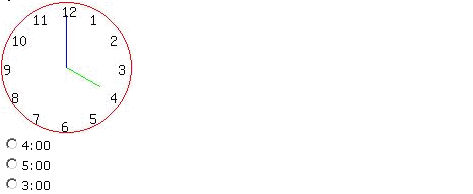Email us to get an instant 20% discount on highly effective K-12 Math & English kwizNET Programs!

#### Online Quiz (WorksheetABCD)

Questions Per Quiz = 2 4 6 8 10

### Grade 2 - Mathematics5.8 Clocks - Time to 15 Minutes (WIZ Math)

 Explanation: A clock contains two hands, one is the hour hand and the other is the minute hand. The shorter hand is the hour hand, the longer hand is the minute hand. When hour hand moves from one number to another number, it takes minute hand one complete circle that is 60 minutes. When minute hand moves from one number to another number, it is 5 minutes 1 hour = 60 minutes. 1/2 hour = 30 minutes 1/4 hour = 15 minutes Method: Look at the time shown by the clock. Write the number that hour hand points to. Put a colon : To write the number that minute hand points to: Count by 5, starting from 12. (12 to 1 it is 5. 1 to 2 another 5, 2 to 3 another 5 and so on....5,10,15,20,25....... ) Time in 15 minutes will be either 15, 30, 45, 00 If the minute hand points to 12 then write 00. Example: What is the time shown in the clock?Answer : 4:00 Directions: Choose the correct time shown in the clock. Also write at least ten examples of your own.
 Q 1: What is the time shown in the clock?3:304:002:003:00 Q 2: What is the time shown in the clock?8:1510:159:459:15 Q 3: What is the time shown in the clock?1:0011:0012:3012:00 Q 4: What is the time shown in the clock?7:457:156:458:45 Q 5: What is the time shown in the clock?12:4511:1510:4511:45 Question 6: This question is available to subscribers only! Question 7: This question is available to subscribers only! Question 8: This question is available to subscribers only! Question 9: This question is available to subscribers only! Question 10: This question is available to subscribers only!